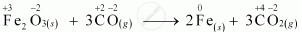CBSE (Science) Class 11CBSE
Share

# Justify that the Following Reactions Are Redox Reactions: Fe2o3(S) + 3co(G) → 2fe(S) + 3co2(G) - CBSE (Science) Class 11 - Chemistry

ConceptOxidation Number Types of Redox Reactions

#### Question

Justify that the following reactions are redox reactions:

Fe2O3(s) + 3CO(g) → 2Fe(s) + 3CO2(g)

#### Solution 1

Fe2O3(s) + 3CO(g) → 2Fe(s) + 3CO2(g)

Let us write the oxidation number of each element in the given reaction as:Here, the oxidation number of Fe decreases from +3 in Fe2O3 to 0 in Fe i.e., Fe2O3 is reduced to Fe. On the other hand, the oxidation number of C increases from +2 in CO to +4 in CO2 i.e., CO is oxidized to CO2. Hence, the given reaction is a redox reaction.

#### Solution 2Here O.N. of Fe decreases from +3 if Fe2O3 to 0 in Fe while that of C increases from +2 in CO to +4 in CO2. Further, oxygen is removed from Fe2O3 and added to CO, therefore, Fe2O3 is reduced while CO is oxidised. Thus, this is a redox reaction.

Is there an error in this question or solution?

#### APPEARS IN

Solution Justify that the Following Reactions Are Redox Reactions: Fe2o3(S) + 3co(G) → 2fe(S) + 3co2(G) Concept: Oxidation Number - Types of Redox Reactions.
S